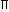# Aptitude - Area

### Exercise :: Area - Data Sufficiency 1

Each of the questions given below consists of a statement and / or a question and two statements numbered I and II given below it. You have to decide whether the data provided in the statement(s) is / are sufficient to answer the given question. Read the both statements and

• Give answer (A) if the data in Statement I alone are sufficient to answer the question, while the data in Statement II alone are not sufficient to answer the question.
• Give answer (B) if the data in Statement II alone are sufficient to answer the question, while the data in Statement I alone are not sufficient to answer the question.
• Give answer (C) if the data either in Statement I or in Statement II alone are sufficient to answer the question.
• Give answer (D) if the data even in both Statements I and II together are not sufficient to answer the question.
• Give answer(E) if the data in both Statements I and II together are necessary to answer the question.
1.

 The area of playground is 1600 m2. What is the perimeter? I. It is a perfect square playground. II. It costs Rs. 3200 to put a fence around the playground at the rate of Rs. 20 per metre.

 A. I alone sufficient while II alone not sufficient to answer B. II alone sufficient while I alone not sufficient to answer C. Either I or II alone sufficient to answer D. Both I and II are not sufficient to answer E. Both I and II are necessary to answer

Explanation:

Area = 1600 m2.

I. Side = 1600 m = 40 m. So, perimeter = (40 x 4) m = 160 m.I alone gives the answer.

 II. Perimeter = Total cost = 3200 m = 160 m. Cost per metre 20II alone gives the answer.Correct answer is (C).

2.

 The area of a rectangle is equal to the area of right-angles triangle. What is the length of the rectangle? I. The base of the triangle is 40 cm. II. The height of the triangle is 50 cm.

 A. I alone sufficient while II alone not sufficient to answer B. II alone sufficient while I alone not sufficient to answer C. Either I or II alone sufficient to answer D. Both I and II are not sufficient to answer E. Both I and II are necessary to answer

Explanation:

Given: Area of rectangle = Area of a right-angles triangle.l x b = 1 x B x H 2

I gives, B = 40 cm.

II gives, H = 50 cm.

Thus, to find l, we need b also, which is not given.Given data is not sufficient to give the answer.Correct answer is (D).

3.

 What is the height of the triangle? I. The area of the triangle is 20 times its base. II. The perimeter of the triangle is equal to the perimeter of a square of side 10 cm.

 A. I alone sufficient while II alone not sufficient to answer B. II alone sufficient while I alone not sufficient to answer C. Either I or II alone sufficient to answer D. Both I and II are not sufficient to answer E. Both I and II are necessary to answer

Explanation:

 I. A = 20 x B1 x B x H = 20 x BH = 40. 2I alone gives the answer.

II gives, perimeter of the triangle = 40 cm.

This does not give the height of the triangle.Correct answer is (A).

4.

 What will be the cost of painting the inner walls of a room if the rate of painting is Rs. 20 per square foot? I. Circumference of the floor is 44 feet. II. The height of the wall of the room is 12 feet.

 A. I alone sufficient while II alone not sufficient to answer B. II alone sufficient while I alone not sufficient to answer C. Either I or II alone sufficient to answer D. Both I and II are not sufficient to answer E. Both I and II are necessary to answer

Explanation:

I gives, 2R = 44.

II gives, H = 12.A = 2RH = (44 x 12).

Cost of painting = Rs. (44 x 12 x 20).

Thus, I and II together give the answer.Correct answer is (E).